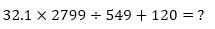IBPS RRB Quantitative Aptitude Quiz

Here, we are providing IBPS RRB Study plan as there is left not enough time to deal in details. The questions asked in the quantitative aptitude section are calculative and very time-consuming. But once dealt with proper strategy, speed, and accuracy, this section can get you the maximum marks in the examination. Following is the Quantitative Aptitude quiz to help you practice with the best of latest pattern questions

Directions (1-15): Find the approximate value of the following questions.

Q1.3050
3780
2340
3420
3950

Q2.1280
2290
1020
18150
1040

Q3.31
9
4
16
21

Q4.311
234
360
290
399

Q5.220
280
375
505
190

Q6. (29.989% of 4530.11) – (22.04% of 4599.99) = ?+ 125.99
289
296
278
221
323

Q7. 14.95% of 679.98 + 19.9% of 219.89 =?
116
146
165
176
85

Q8. 820.01 – 21 × 32.99 +? = 240
105
173
113
234
143

Q9. 8537.986 – 2416.005 – 221.996 =?
6500
5900
4300
3900
5050

Q10. 2489.99 ÷ 9.97 + 54.94% of 279.95 =?
373
445
507
302
403

Q11. 1011.11 + 110.1 + 111.01 =?
1230
1300
1130
1070
1700

Q12. 12.005% of 624.999 =?
91
58
62
75
80

Q13. 16.007 × 14.995 × 6.080 =?
1510
1440
1200
1330
1480

Q14. 7000.001 ÷ 699.983 × 4.020 =?
58
32
60
40
50

Q15. 23.999 × 9.004 × 16.997 =?
3200
4100
2700
3670
3400

You May also like to Read: